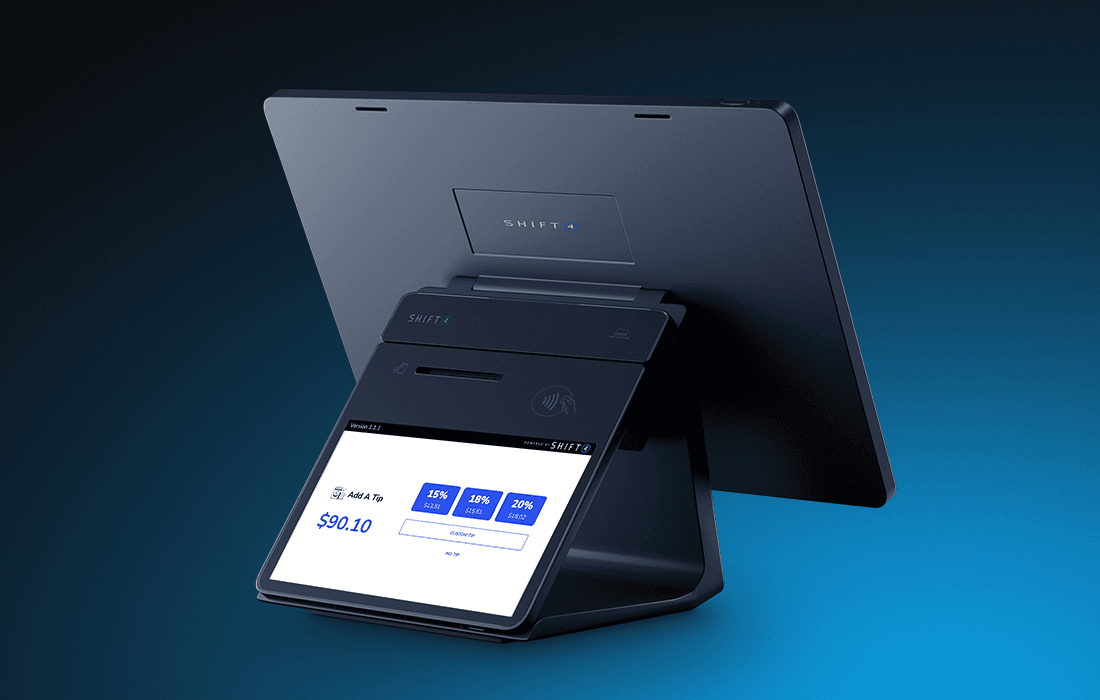# POS Hardware Built for Restaurantsself.__wrap_balancer=(t,e,n)=>{n=n||document.querySelector(`[data-br="\${t}"]`);let o=n.parentElement,r=E=>n.style.maxWidth=E+"px";n.style.maxWidth="";let i=o.clientWidth,s=o.clientHeight,c=i/2,u=i,d;if(i){for(;c+1<u;)d=~~((c+u)/2),r(d),o.clientHeight==s?u=d:c=d;r(u*e+i*(1-e))}};self.__wrap_balancer(":R19lm6:",1)

Designed to take the heat from the kitchen, our point of sale hardware is reliable, durable, and comes with a lifetime warranty.## SkyTab POSself.__wrap_balancer=(t,e,n)=>{n=n||document.querySelector(`[data-br="\${t}"]`);let o=n.parentElement,r=E=>n.style.maxWidth=E+"px";n.style.maxWidth="";let i=o.clientWidth,s=o.clientHeight,c=i/2,u=i,d;if(i){for(;c+1<u;)d=~~((c+u)/2),r(d),o.clientHeight==s?u=d:c=d;r(u*e+i*(1-e))}};self.__wrap_balancer(":R5ilm6:",1)

Power your restaurant with next generation POS

• Flawless performance
• Modern, sleek design
• Integrated customer display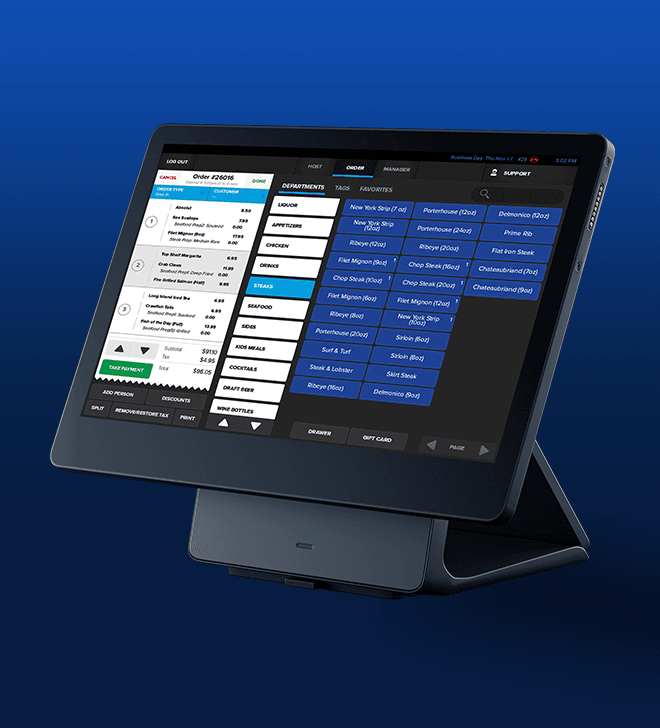## SkyTab Mobileself.__wrap_balancer=(t,e,n)=>{n=n||document.querySelector(`[data-br="\${t}"]`);let o=n.parentElement,r=E=>n.style.maxWidth=E+"px";n.style.maxWidth="";let i=o.clientWidth,s=o.clientHeight,c=i/2,u=i,d;if(i){for(;c+1<u;)d=~~((c+u)/2),r(d),o.clientHeight==s?u=d:c=d;r(u*e+i*(1-e))}};self.__wrap_balancer(":R5jlm6:",1)

Delight your guests from tableside to curbside

• Accept payments anywhere
• Mobile ordering capabilities
• Built-in customer survey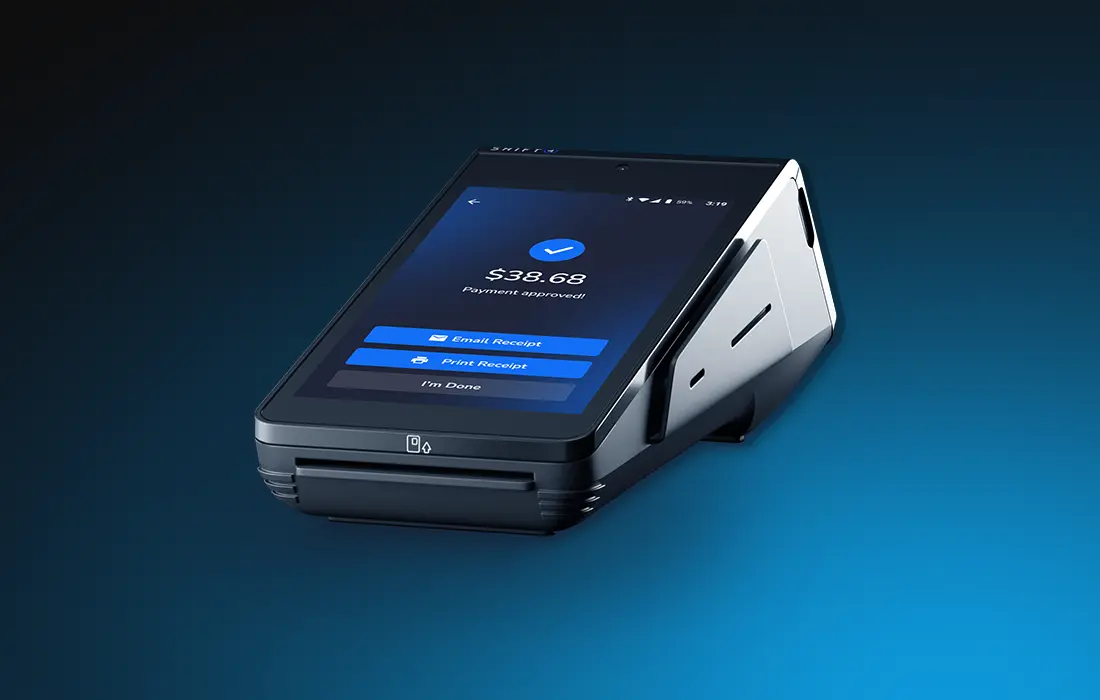## SkyTab Glassself.__wrap_balancer=(t,e,n)=>{n=n||document.querySelector(`[data-br="\${t}"]`);let o=n.parentElement,r=E=>n.style.maxWidth=E+"px";n.style.maxWidth="";let i=o.clientWidth,s=o.clientHeight,c=i/2,u=i,d;if(i){for(;c+1<u;)d=~~((c+u)/2),r(d),o.clientHeight==s?u=d:c=d;r(u*e+i*(1-e))}};self.__wrap_balancer(":R5klm6:",1)

Supercharge your servers with fully mobile POS

• Purpose-built hardware
• Complete POS software
• Optimal 8” screen size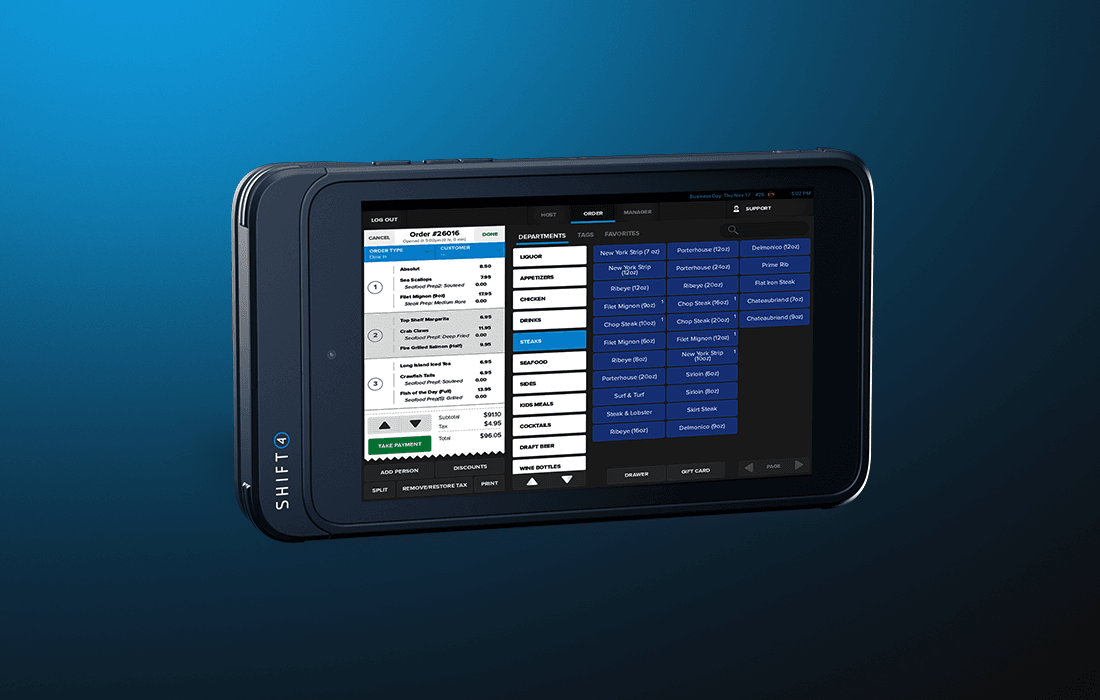## Kitchen Display Systemself.__wrap_balancer=(t,e,n)=>{n=n||document.querySelector(`[data-br="\${t}"]`);let o=n.parentElement,r=E=>n.style.maxWidth=E+"px";n.style.maxWidth="";let i=o.clientWidth,s=o.clientHeight,c=i/2,u=i,d;if(i){for(;c+1<u;)d=~~((c+u)/2),r(d),o.clientHeight==s?u=d:c=d;r(u*e+i*(1-e))}};self.__wrap_balancer(":R5llm6:",1)

Optimize operations by connecting FOH to BOH

• Intuitive interface
• Large 22” display
• Touchscreen controls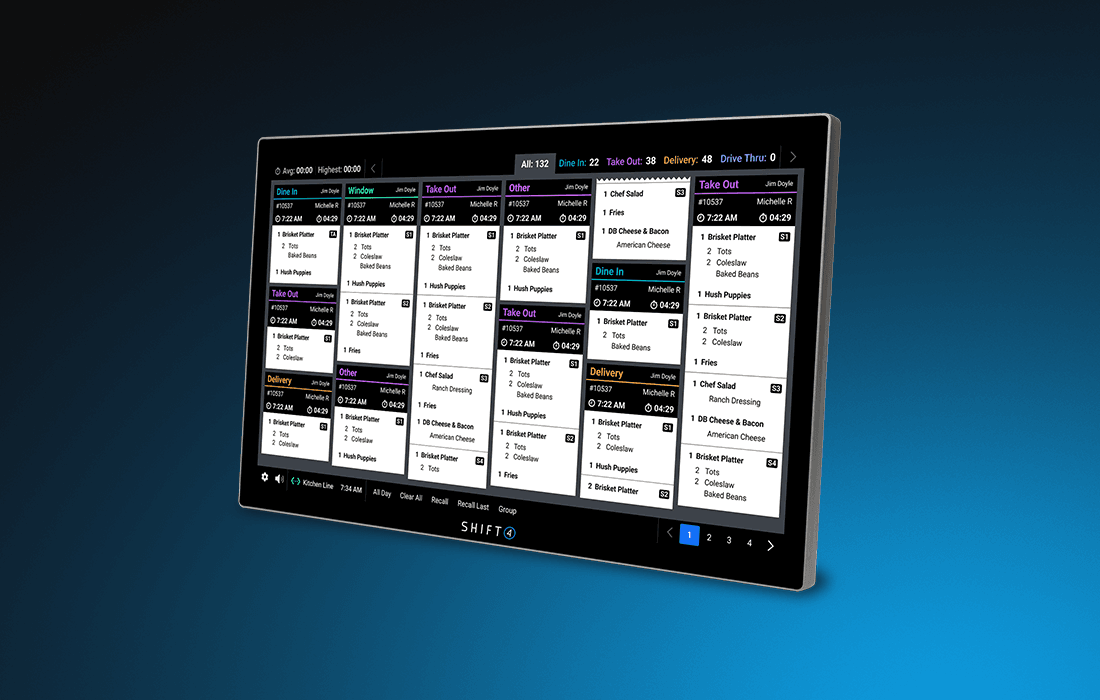## SkyTab Customer-Facing Displayself.__wrap_balancer=(t,e,n)=>{n=n||document.querySelector(`[data-br="\${t}"]`);let o=n.parentElement,r=E=>n.style.maxWidth=E+"px";n.style.maxWidth="";let i=o.clientWidth,s=o.clientHeight,c=i/2,u=i,d;if(i){for(;c+1<u;)d=~~((c+u)/2),r(d),o.clientHeight==s?u=d:c=d;r(u*e+i*(1-e))}};self.__wrap_balancer(":R5mlm6:",1)

Engage guests and increase tips

• Improved order accuracy
• Built-in loyalty program
• Pre-set gratuity prompts